In [ ]:
%matplotlib inline
from fastai.basics import *


## MNIST SGD¶

Get the 'pickled' MNIST dataset from http://deeplearning.net/data/mnist/mnist.pkl.gz. We're going to treat it as a standard flat dataset with fully connected layers, rather than using a CNN.

In [ ]:
path = Config().data_path()/'mnist'

In [ ]:
path.ls()

Out[ ]:
[PosixPath('/home/ubuntu/.fastai/data/mnist/mnist.pkl.gz')]
In [ ]:
with gzip.open(path/'mnist.pkl.gz', 'rb') as f:
((x_train, y_train), (x_valid, y_valid), _) = pickle.load(f, encoding='latin-1')

In [ ]:
plt.imshow(x_train.reshape((28,28)), cmap="gray")
x_train.shape

Out[ ]:
(50000, 784)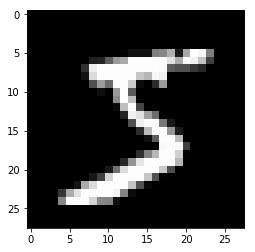In [ ]:
x_train,y_train,x_valid,y_valid = map(torch.tensor, (x_train,y_train,x_valid,y_valid))
n,c = x_train.shape
x_train.shape, y_train.min(), y_train.max()

Out[ ]:
(torch.Size([50000, 784]), tensor(0), tensor(9))

In lesson2-sgd we did these things ourselves:

x = torch.ones(n,2)
def mse(y_hat, y): return ((y_hat-y)**2).mean()
y_hat = x@a


Now instead we'll use PyTorch's functions to do it for us, and also to handle mini-batches (which we didn't do last time, since our dataset was so small).

In [ ]:
bs=64
train_ds = TensorDataset(x_train, y_train)
valid_ds = TensorDataset(x_valid, y_valid)
data = DataBunch.create(train_ds, valid_ds, bs=bs)

In [ ]:
x,y = next(iter(data.train_dl))
x.shape,y.shape

Out[ ]:
(torch.Size([64, 784]), torch.Size())
In [ ]:
class Mnist_Logistic(nn.Module):
def __init__(self):
super().__init__()
self.lin = nn.Linear(784, 10, bias=True)

def forward(self, xb): return self.lin(xb)

In [ ]:
model = Mnist_Logistic().cuda()

In [ ]:
model

Out[ ]:
Mnist_Logistic(
(lin): Linear(in_features=784, out_features=10, bias=True)
)
In [ ]:
model.lin

Out[ ]:
Linear(in_features=784, out_features=10, bias=True)
In [ ]:
model(x).shape

Out[ ]:
torch.Size([64, 10])
In [ ]:
[p.shape for p in model.parameters()]

Out[ ]:
[torch.Size([10, 784]), torch.Size()]
In [ ]:
lr=2e-2

In [ ]:
loss_func = nn.CrossEntropyLoss()

In [ ]:
def update(x,y,lr):
wd = 1e-5
y_hat = model(x)
# weight decay
w2 = 0.
for p in model.parameters(): w2 += (p**2).sum()
loss = loss_func(y_hat, y) + w2*wd
loss.backward()
for p in model.parameters():
return loss.item()

In [ ]:
losses = [update(x,y,lr) for x,y in data.train_dl]

In [ ]:
plt.plot(losses);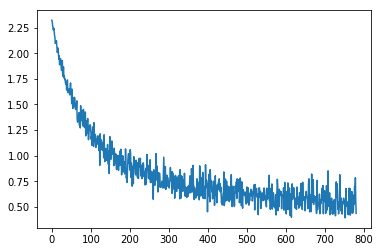In [ ]:
class Mnist_NN(nn.Module):
def __init__(self):
super().__init__()
self.lin1 = nn.Linear(784, 50, bias=True)
self.lin2 = nn.Linear(50, 10, bias=True)

def forward(self, xb):
x = self.lin1(xb)
x = F.relu(x)
return self.lin2(x)

In [ ]:
model = Mnist_NN().cuda()

In [ ]:
losses = [update(x,y,lr) for x,y in data.train_dl]

In [ ]:
plt.plot(losses);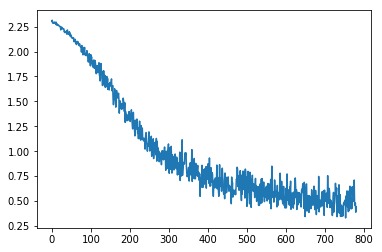In [ ]:
model = Mnist_NN().cuda()

In [ ]:
def update(x,y,lr):
y_hat = model(x)
loss = loss_func(y_hat, y)
loss.backward()
opt.step()
return loss.item()

In [ ]:
losses = [update(x,y,1e-3) for x,y in data.train_dl]

In [ ]:
plt.plot(losses);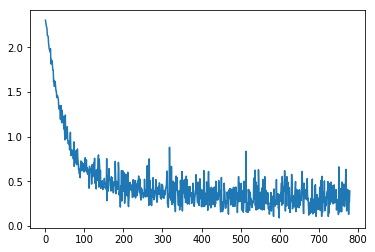In [ ]:
learn = Learner(data, Mnist_NN(), loss_func=loss_func, metrics=accuracy)

---------------------------------------------------------------------------
AttributeError                            Traceback (most recent call last)
<ipython-input-28-7aa74a9f84cb> in <module>
----> 1 learn = Learner(data, Mnist_NN(), loss_func=loss_func, metrics=accuracy)

<string> in __init__(self, data, model, opt_func, loss_func, metrics, true_wd, bn_wd, wd, train_bn, path, model_dir, callback_fns, callbacks, layer_groups, add_time)

~/fastai/fastai/basic_train.py in __post_init__(self)
154         (self.path/self.model_dir).mkdir(parents=True, exist_ok=True)
155         self.model = self.model.to(self.data.device)
--> 156         self.loss_func = ifnone(self.loss_func, self.data.loss_func)
157         self.metrics=listify(self.metrics)
158         if not self.layer_groups: self.layer_groups = [nn.Sequential(*flatten_model(self.model))]

~/fastai/fastai/basic_data.py in __getattr__(self, k)
120         return cls(*dls, path=path, device=device, dl_tfms=dl_tfms, collate_fn=collate_fn, no_check=no_check)
121
--> 122     def __getattr__(self,k:int)->Any: return getattr(self.train_dl, k)
123     def __setstate__(self,data:Any): self.__dict__.update(data)
124

~/fastai/fastai/basic_data.py in __getattr__(self, k)
36
37     def __len__(self)->int: return len(self.dl)
---> 38     def __getattr__(self,k:str)->Any: return getattr(self.dl, k)
39     def __setstate__(self,data:Any): self.__dict__.update(data)
40

19
---> 20 def DataLoader___getattr__(dl, k:str)->Any: return getattr(dl.dataset, k)
22

AttributeError: 'TensorDataset' object has no attribute 'loss_func'
In [ ]:
%debug

> /home/ubuntu/fastai/fastai/basic_data.py(20)DataLoader___getattr__()
19
---> 20 def DataLoader___getattr__(dl, k:str)->Any: return getattr(dl.dataset, k)
22

ipdb> u
> /home/ubuntu/fastai/fastai/basic_data.py(38)__getattr__()
36
37     def __len__(self)->int: return len(self.dl)
---> 38     def __getattr__(self,k:str)->Any: return getattr(self.dl, k)
39     def __setstate__(self,data:Any): self.__dict__.update(data)
40

ipdb> print(k)
loss_func
ipdb> q

In [ ]:
learn.lr_find()
learn.recorder.plot()

LR Finder is complete, type {learner_name}.recorder.plot() to see the graph.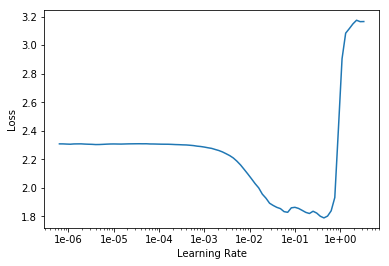In [ ]:
learn.fit_one_cycle(1, 1e-2)

Total time: 00:03

epoch train_loss valid_loss accuracy
1 0.129131 0.125927 0.963500
In [ ]:
learn.recorder.plot_lr(show_moms=True)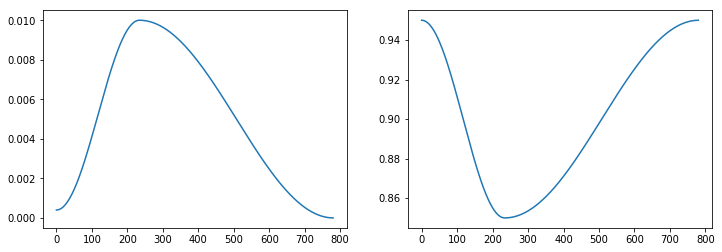In [ ]:
learn.recorder.plot_losses()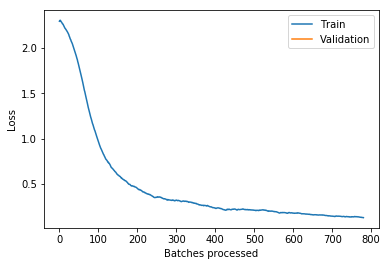## fin¶

In [ ]: